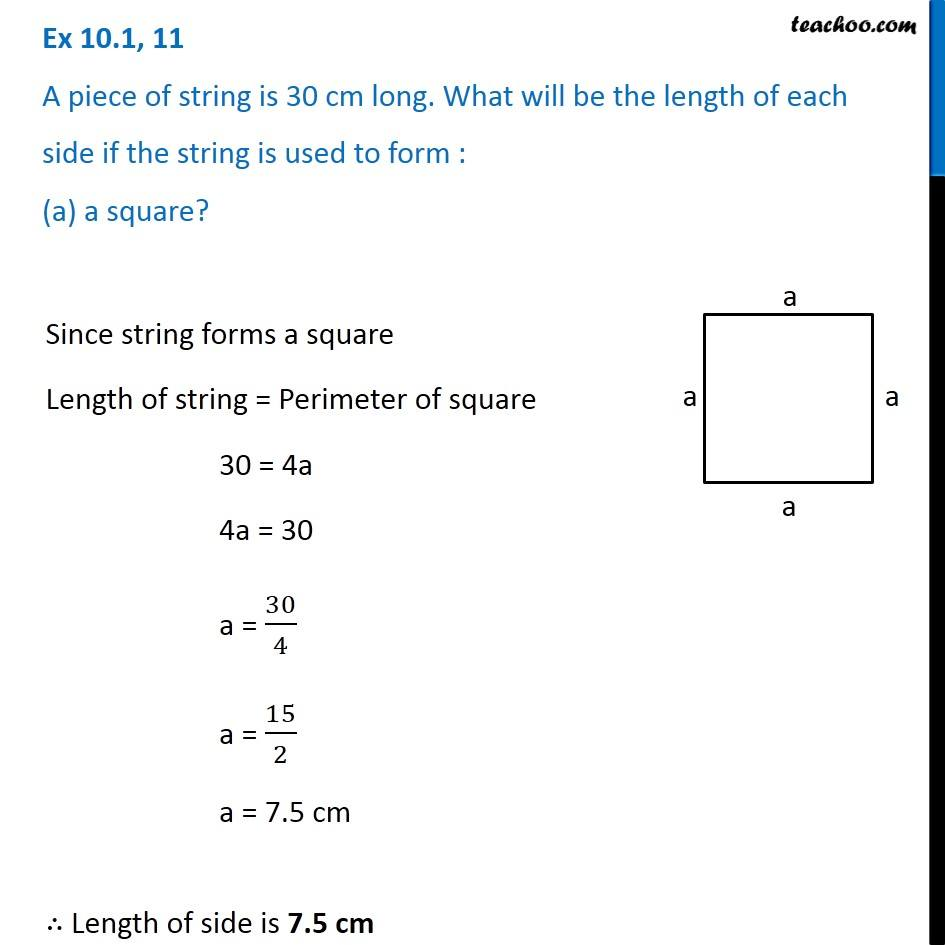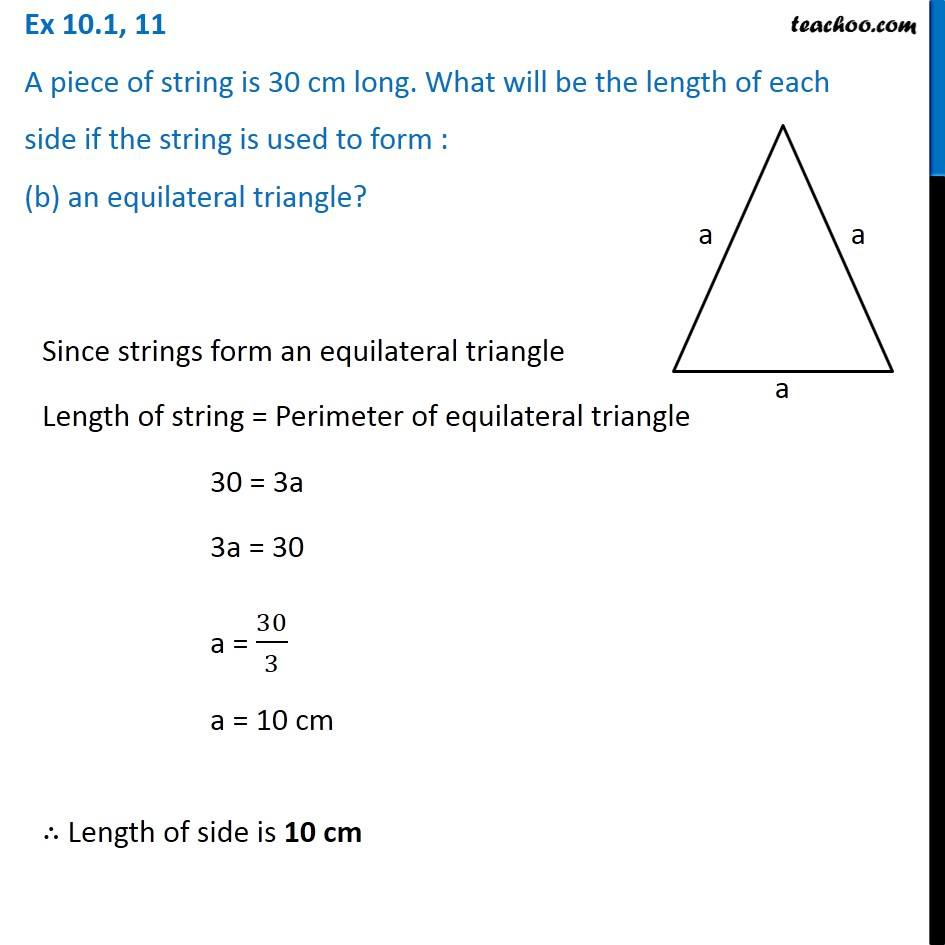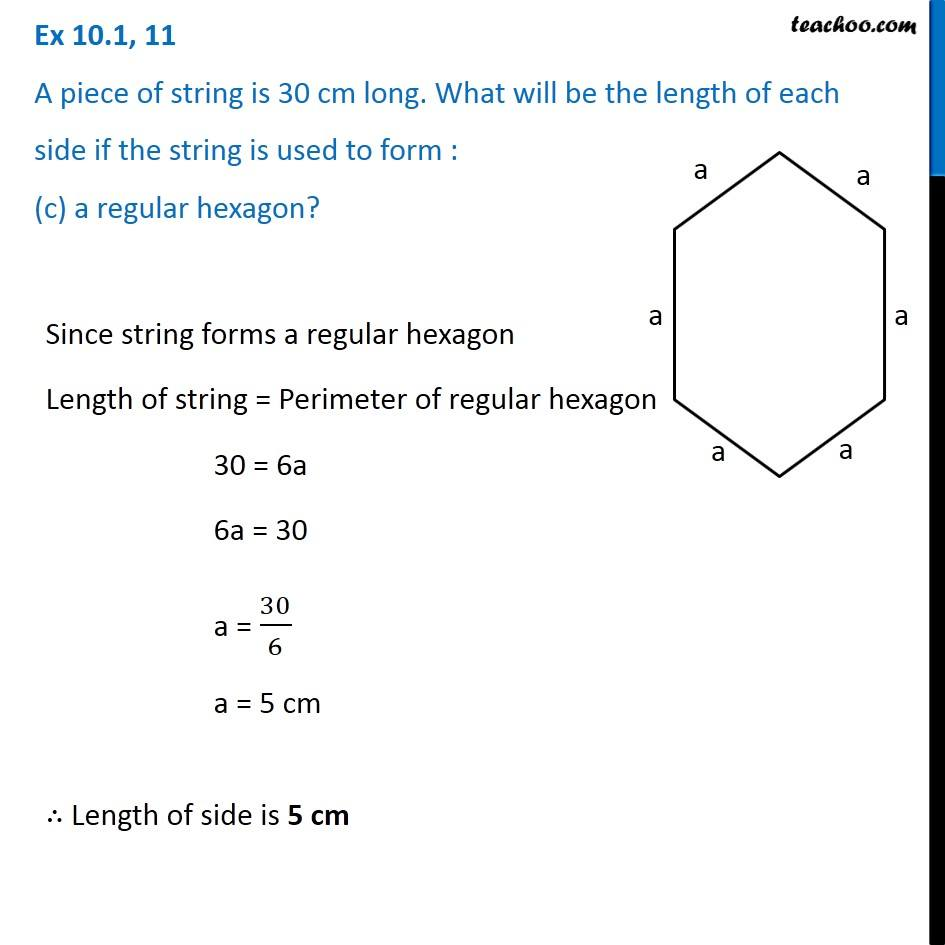1. Chapter 10 Class 6 Mensuration
2. Serial order wise
3. Ex 10.1

Transcript

Ex 10.1, 11 A piece of string is 30 cm long. What will be the length of each side if the string is used to form : (a) a square? Since string forms a square Length of string = Perimeter of square 30 = 4a 4a = 30 a = 30/4 a = 15/2 a = 7.5 cm ∴ Length of side is 7.5 cm Ex 10.1, 11 A piece of string is 30 cm long. What will be the length of each side if the string is used to form : (b) an equilateral triangle? Since strings form an equilateral triangle Length of string = Perimeter of equilateral triangle 30 = 3a 3a = 30 a = 30/3 a = 10 cm ∴ Length of side is 10 cm Ex 10.1, 11 A piece of string is 30 cm long. What will be the length of each side if the string is used to form : (c) a regular hexagon? Since string forms a regular hexagon Length of string = Perimeter of regular hexagon 30 = 6a 6a = 30 a = 30/6 a = 5 cm ∴ Length of side is 5 cm

Ex 10.1